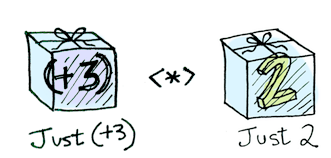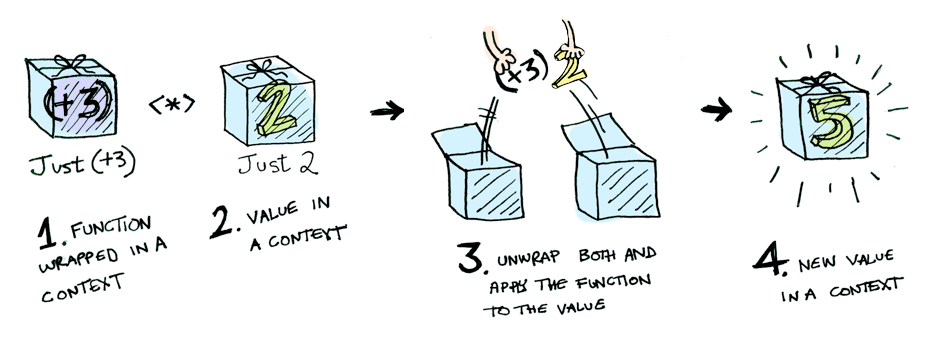# [Day06] Applicative

``````fmap :: (Functor f) -> (a -> b) -> f a -> f b
````````````class Functor f => Applicative f where
pure :: a -> f a
(<*>) :: f (a -> b) -> f a -> f b
``````

`<*>` 這個運算子(fun fact：運算子也是函數)的作用就是將在 context 中的函數 map over 到在 context 中的 value，我們比較一下 `fmap``<*>`

``````fmap :: Functor f => (a -> b) -> f a -> f b
<*> :: Functor f => f (a -> b) -> f a -> f b
````````````const callback = new Promise(...)
const value = new Promise(...)
value.then(callback)
``````

* 本文圖片來自這裏

#Functional Programming #程式設計